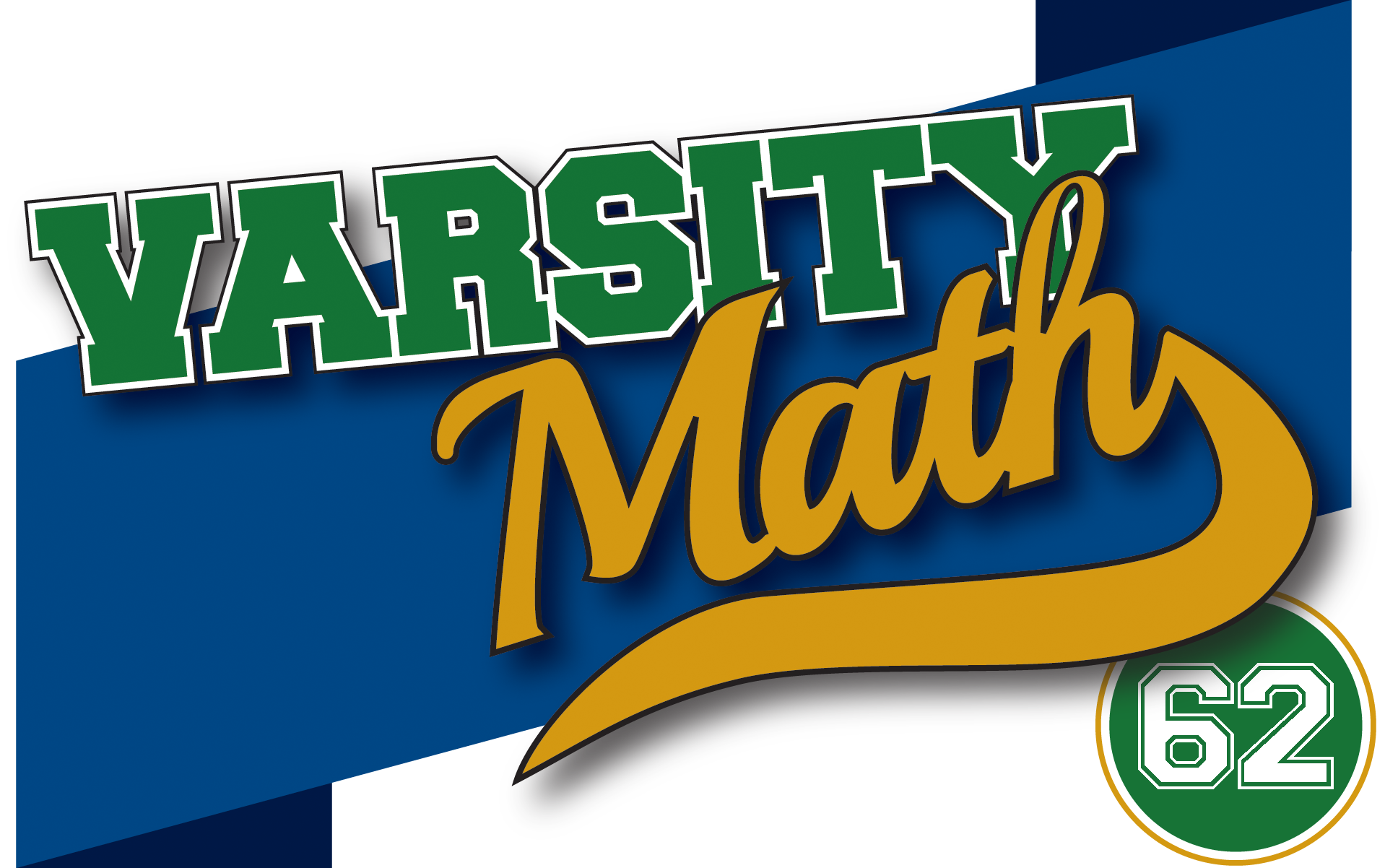## ________________

Although their university’s focus is on the gridiron this time of year, the Varsity Math team’s focus this week is just on the grid.

## ________________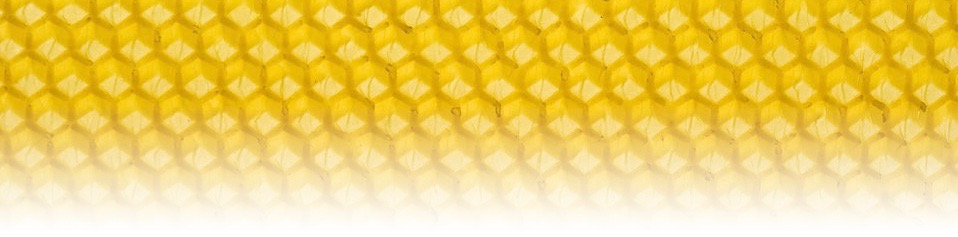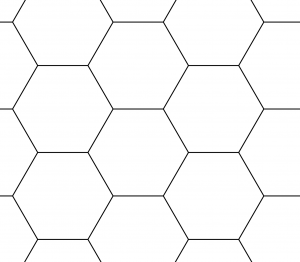Kai is messing around with various grids, and stumbles upon this interesting coincidence on a hexagonal grid. A, B, and C are three different grid points such that A and C are both connected by a unit-length grid line to B. Kai draws a, the circle with center A that goes through C, and b, the circle with center B through C. The point where the ray from B through A intersects a, Kai calls D. Kai also labels as E the other point where line DC intersects b.

What is angle EBC?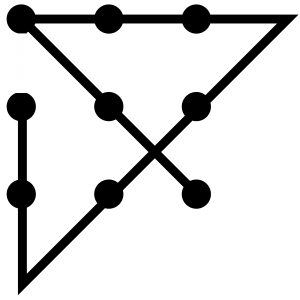### Way Outside the Box

Coach Newton is giving a problem-solving lecture and covers two interesting principles. First, a good way to create new problems is to extend old problems, sometimes just by changing one of the quantities. Second, it is important to think outside the box. The Coach points out that the phrase “outside the box” may itself come from a classic problem: to draw a continuous line connecting each of the nine points in a 3-by-3 grid with the fewest line segments. (The solution, at left, explains the phrase.) But, Coach Newton concludes, it’s not too common for people to combine these two principles, so let’s give it a try right now.

What is the fewest number of line segments connected end to end that pass through each of the 16 points in a 4-by-4 grid, so that each point lies on exactly one of the line segments, and so that by deleting the first line segment, the remaining lines pass through all twelve of the points in a 3-by-4 grid?

## Solutions to week 61

Further Fairness.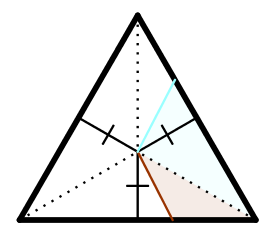Let’s re-examine why the center of the equilateral triangle in the previous week’s Edible Division worked as a point to cut to, that would ensure that slices with equal perimeter have equal area. It’s because that point divides the triangle into three triangles of the same height, and so any slice cut out of the cake (say by the red and blue cuts in the diagram) consists of (triangular) portions of one or two of those triangles, and the area of each portion is 1/2 times that common height times the portion of of the base (perimeter) cut out. So (possibly adding up two portions) the area of any slice from the center is just 1/2 times the common height times the amount of perimeter included.Therefore the question becomes: is there any point inside a 3-4-5 right triangle which is the same “height” from all three sides? If so, we can cut to that point and the exact same argument will show that equal-perimeter slices are equal-area slices. What does it mean for a point to be a certain “height” from a line segment? It means that the perpendicular from that point to the line segment has a given length. But note as in the next diagram that if you draw a perpendicular from a point to a line segment, the line segment is tangent to a circle centered at that point. And it works the other way around, too: if a circle is tangent to a line segment, then the radius from the center of the point to the point of tangency is perpendicular to the line segment.The upshot of all of that is that the answer is yes: the center of the inscribed circle of the 3-4-5 triangle is the same height from all three sides, and so will serve as the “cake-cutting center” of the triangle as asked for in this problem.

Now, you might not be satisfied quite yet; you might reasonably ask, “Yes, but where is that center of the inscribed circle?” There are a couple of ways to answer that question. First, you might simply recall that the center of the inscribed circle is the intersection of the angle bisectors of the triangle, as shown. So to find the “cutting center” of an actual triangular cake, you would just bisect the angles at two of the vertices and take the point where the bisectors cross. Or you might be thinking in terms of coordinates. If you put the 3-4-5 triangle down on a coordinate plane in the most common way as shown, what are the coordinates of the center of the inscribed circle? There are multiple ways to solve that problem. The most direct is first to notice that the angle bisector of the right angle is just the diagonal of the coordinate plane, so the desired point has coordinates (c,c) for some value c. Also it is clear that the perpendicular distance of the point (c,c) to each of the legs (since they are on the axes) is just c. So this method would be to find the equation of the line which is the hypotenuse (which is easy because we know the slope and y-intercept, so it is y = -3x/4 + 3), find the equation of the line perpendicular to the hypotenuse through (c,c) (also fairly easy; the slope is 4/3, so point-slope form tells you it is y-c = 4/3(x-c), or y = 4x/3 – c/3), then find the coordinates of the point where that perpendicular and the hypotenuse intersect (set y-values in the two equations equal to each other and solve for x, i.e. -3x/4 + 3 = 4x/3 – c/3, so 3 + c/3 = 25x/12, or x = (36 + 4c)/25, and then y = (144 – 9c)/75), calculate the square of the distance from (c,c) to that intersection point (it’s (36-21c)²/625 + (48-28c)²/625), and set that equal to c², and solve for c. (I’ll let you do that!)

But sometimes the most direct way is not the least work. A second approach would be to figure out the slope of the angle bisector at point A. The tangent of the angle at A is the opposite side over the adjacent side, or just 3/4, which is the negative of the slope of the hypotenuse. Using the tangent half-angle formula, the tangent of the angle A/2 = tan A/(1 + 1/cos A). Noting that cosine is the adjacent over the hypotenuse, or 4/5, we get that tan A/2 = 3/4 / (1+5/4) = 3/4 / 9/4 = 1/3. So the slope of the angle bisector at A is -1/3, and the equation of that line is y = –x/3 + 4/3. Now you just need to solve for the intersection point of the diagonal y = x and this other bisector line to find the coordinates of the center of the inscribed circle, which is a lot less work (and which again I will let you do).

However, the easiest way by far to find the coordinates of the inscribed circle is to use the formula for the center of the inscribed circle in barycentric coordinates, which is just a : b : c = 3 : 4 : 5 = (3(4,0) + 4(0,3) + 5(0,0))/(3+4+5) = ((12,0) + (0,12))/12 = (1,1). That’s right, the coordinates of the center of the inscribed circle of the 3-4-5 triangle placed on the axes as shown are just (1,1)! If you didn’t already do all of the algebra in the previous two methods, you can just verify that substituting c = 1 in the first method makes the final equation hold, or that the point (1,1) is indeed on both lines in the second method (it is clearly on y = x). So now we know exactly how to cut the cake, as students at Borough of Manhattan Community College recently learned as well.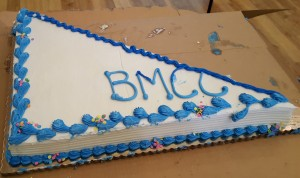Coach Newton expounds further:
This problem actually goes much deeper. Clearly any triangle has an inscribed circle, and so can be cut in this way. Does any shape have a point from which it can be cut so that equal perimeter yields equal area? No; the easiest shape to see this is with a long, skinny rectangle. No matter where you try to locate a “cutting center,” it will be different distances to a long side and one or the other of the short sides, and so slices with equal bases cut out of just those sides will have different areas. On the other hand, you can cut to the center of aregular pentagon, say, because it does have an inscribed circle. So the natural question is, what are all of the shapes that have a “cake-cutting center” so that equal-perimeter slices to that center have equal area? This becomes a question of calculus, because you need that any tiny slice at any point has area proportional to the bit of perimeter, a.k.a. “arc length,” cut off. If you put the cutting center at the origin, that leads to a polar differential equation for the curve following the perimeter of the cake, relating the area of a slice and the arc length cut off, namely r²/2 = k√(r² + (dr/)²). It turns out that this differential equation has only two solutions, circular arcs and straight lines, leading to the conclusion that any cake which has a “cutting center” consists of circular arcs and tangent lines, all of the same circle. That still allows some pretty funny-shaped cakes, as in this example.

Double-Crossed Training. Clearly we want the “slow rowers” to be in the boat with the 1-minute and 2-minute rowers so that the crossing only takes two minutes. That suggests the following scheme.So the students can definitely cross in nine minutes. Can they possibly do it any more quickly? If the boat goes back and forth any more times, since in each round trip only one of the two directions can take one minute (the 1-minute rower alone or just the 1- and 2-minute rowers) in order to drop anyone else off on the far side, there will be at least three two-minute trips and at least three one-minute trips, for at least nine minutes. Since there are six passengers, the boat must go back and forth at least three times. But if both the 1-minute and 2-minute rowers cross each time, then there is not enough capacity left in the boat to get everyone across in just three trips. So we conclude that there must be at least one trip in which the the 2-minute rower is not present, but a slower rower is. That trip will take at least three minutes, and as we noted earlier, the other trips can do no better than alternating one and two minutes. Hence the students need at least 9 minutes to get across.

## Recent Weeks

Links to all of the puzzles and solutions are on the Complete Varsity Math page.

Come back next week for answers and more puzzles.

[asciimathsf]